# Important 5 Marks Questions for CBSE 11 Chemistry Exam

Class 11 chemistry important 5 mark questions with answers are provided here. These important questions are based on CBSE board curriculum and correspond to the most recent Class 11 chemistry syllabus. By practising these Class 11 important 5 mark questions, students will be able to quickly review all of the ideas covered in the chapter and prepare for the Class 11 Annual examinations as well as other entrance exams such as NEET and JEE.

Download Class 11 Chemistry Important 5 Mark Questions with Answers PDF by clicking on the button below.

## Class 11 Chemistry Important 5 Mark Questions with Answers

Q1:

Explain the following reactions:

1. Silicon is heated with methyl chloride at a high temperature in the presence of copper powder
2. CO is heated with ZnO
3. The reaction of boron trifluoride with LiAlH4 in diethyl ether
4. The reaction of boron trifluoride with sodium hydride at 450 K
5. The reaction of diborane and water

1. Silicon is heated with methyl chloride at a high temperature in the presence of copper powder

A combination of mono-di and trimethyl chlorosilanes, as well as a minor quantity of tetramethylsilane, is generated when silicon combines with methyl chloride in the presence of copper at a high temperature (such as 537K). Methyl substituted chlorosilanes MeSiCl3, Me2SiCl2, Me3SiCl and Me4Si are formed.

$$\begin{array}{l}CH_{3}Cl + Si \xrightarrow[537K]{Cu powder} CH_{3}SiCl_{3} + (CH_{3})_{2}SiCl_{2} + (CH_{3})_{3}SiCl + (CH_{3})_{4}Si\end{array}$$

1. CO is heated with ZnO

CO is heated with ZnO because CO is a reducing agent that reduces ZnO to Zn when it reacts with it.

ZnO + CO → Zn + CO2

1. The reaction of boron trifluoride with LiAlH4 in diethyl ether

This reaction is the Preparation of Diborane (B2H6).

4BF4 + 3LiAlH4 → 2B2H6 + LiF + 3AlF3

1. The reaction of boron trifluoride with sodium hydride at 450 K

When Boron trifluoride is heated with Sodium Hydride at 450 K, Diborane is formed. This is how Diborane is made in the industrial world.

$$\begin{array}{l}2BF_{3} + 6NaH \xrightarrow[]{450 K} B_{2}H_{6} + 6NaF\end{array}$$

1. The reaction of diborane and water

Diborane is a flexible and highly reactive reagent with a wide range of uses. It forms hydrogen and boric acid when it reacts forcefully with water:

B2H6 + 6H2O → 2B(OH)3 + 6H2

Q2:

Explain giving reasons for the following:

1. Boron does not form B3+ ions.
2. Molten aluminium bromide is a poor conductor of electricity.
3. BCl3 is more stable than TlCl3.
4. B-Cl bond has a dipole moment but BCl3 has zero dipole moment.
5. Al is used to make transmission cables.

1. Due to the boron atom’s small size, a substantial amount of energy is required to remove three electrons. As a result, Boron does not form the B3+ ion.

Boron has an atomic number of 5. Its electronic configuration is 1s2s2p.

When one electron from the p orbital is removed, He-like fulfilled s orbital is left. This is a really stable situation. As a result, the enthalpy of second ionisation is relatively large. When one electron is withdrawn, a half-filled orbital is created once more. As a result, the third ionisation enthalpy is rather high as well.

Because the total energy required to manufacture B3+ is equal to the sum of all ionisation enthalpies, it takes a tremendous amount of energy to create it.

1. Al2Br6 is a dimer of aluminium bromide. Each aluminium atom accepts a lone pair of electrons from the bromine atom of another aluminium bromide molecule to make one coordinate bond, completing an octet of electrons. Molten aluminium bromide is a poor conductor of electricity due to the absence of free electrons.
2. The periodic table group 13 includes boron and thallium. As you progress down the group, the +1 oxidation state becomes more stable. Because B’s +3 oxidation state is more stable than Tl’s +3 oxidation state, BCl3 is more stable than TlCl3. The +3 form of Tl is strongly oxidising, and it quickly reverts to the more stable +1 state.
3. Because the electronegativity of boron and chlorine atoms differs, B-Cl has a dipole. A molecule’s total dipole is also influenced by its geometry. BCl3 has a planar shape with a bond angle of 120 degrees. The dipole formed by two B-Cl bonds cancels out the third, resulting in a net-zero dipole.
4. Transmission cables are made of aluminium wire. Aluminium wires are good electrical conductors, as well as a cheap metal that is readily available, light in weight, and ductile. Aluminium’s characteristics make it ideal for transmission cable production.

Q3:

For the reaction NH4Cl s-> NH3 g + HCl g at 25°C, enthalpy change ΔH = +177 kJ mol-1 and entropy change ΔS = +285JK-1 mol-1 . Calculate the free energy change of ΔG at 25 C and predict whether the reaction is spontaneous or not.

Given, ΔH = +177 kJ mol-1, ΔS = +285JK-1 mol-1

T = 298 K

Using the relation, ΔG = ΔH – TΔS

ΔG = (177*103 J mol-1) – (298 K)(285 JK-1 mol-1)

ΔG = 92070 J/mole

i.e., ΔG = 92.07 kJ/mole

Thus, ΔG > 0

The reaction is nonspontaneous since G is positive.

Q4:

(a) Compound ‘A’ with the molecular formula C5H8 reacts with hydrogen in the presence of Lindlar’s catalyst to form a compound B with the molecular formula C5 H10. A on reacting with sodium in liquid ammonia forms a compound ‘C’ with the same molecular formula as that of B. Identify ‘A’, ‘B’ and ‘C’. Give the chemical reactions involved.

(b) Write the chemical reaction involved in Kolbe’s electrolytic process. What are the products formed at cathode and anode?

(a) Compound ‘A’ with the molecular formula C5H8 reacts with hydrogen in the presence of Lindlar’s catalyst to form a compound ‘B’ with the molecular formula C5H10. ‘A’ on reacting with sodium in liquid ammonia forms a compound ‘C’ with the same molecular formula as that of ‘B’

Compound A produces cis isomer when it reacts with hydrogen in the presence of Lindlar’s catalyst (Pd/ C).

R1-C≡C-R2 + H2 & Lindlar catalyst → cis R1-CH=CH-R2

When compound A reacts with sodium in liquid ammonia, the trans isomer is formed.

R1-C≡C-R2 + 2Na in NH3 (liq) → trans R1-CH=CH-R2 + 2NaNH2

Here, R1=CH3CH2 and R2=CH3

A = CH3CH2CCCH3 and B = CH3CH2CHCHCH3 and C = CH3CH2CHCHCH3

(b) Kolbe’s electrolysis method produces the appropriate alkane from the sodium salt of fatty acid. The decarboxylation of the sodium salt of fatty acid occurs in this process.

2CH3COONa + 2H2O → CH3 – CH3 + 2CO2 + H2 + 2NaOH

Electrolysis of two molecules of sodium acetate and two molecules of water yields one molecule of ethane, two molecules of carbon dioxide, one molecule of hydrogen, and two molecules of sodium hydroxide in this process.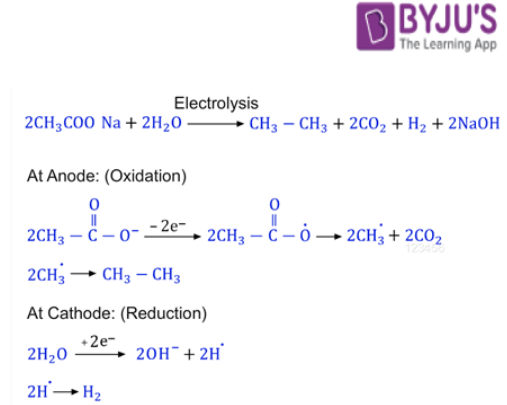Water molecules break down near the cathode, forming hydrogen and hydroxyl ions. A hydrogen molecule is formed when two hydrogen ions unite to produce a gas. Sodium acetate molecules initially create acetate and sodium ions at the anode.

After that, two acetate ions receive two electrons, resulting in two acetate free radicals. Further electrolysis produces two methyl free radicals and two carbon dioxide molecules, which are expelled as a gas.

Q5:

Define the compressibility factor? Explain how the function PV/RT (Z) can be used to show gases behave non-ideally at different pressures.

The gas deviation factor, often known as the compressibility factor Z, is a modifying factor for actual gases. It is the ratio of the volume of a gas at a given temperature and pressure to the volume that the gas would occupy if it were an ideal gas at the same temperature and pressure.

The number of moles of an ideal gas in the sample is equal to the PV/RT ratio. For all pressure, volume, and temperature conditions, this number should remain constant. The gas sample is not behaving optimally if the value of this ratio changes with increasing pressure.

Q6:

Explain the classification of elements into ‘s,p,d & f’ block elements. Explain with suitable examples. Write their general electronic configuration.

Hint: Consider the elements that are located in different blocks of the periodic table and examine them so that we may gain a sense of how the s, p, d, and f-block elements are classified in the periodic table.

In the long form of the periodic table, the elements are divided into four groups. The s, p, d, and f-block elements are the ones in question. The name of the orbitals that receive the last electron is used to classify them.

S-block elements are those in which the valence shell electron reaches the s-orbital. Let’s use sodium as an example. 1s22s22p63s1 is the electronic configuration of sodium. We can deduce from the electronic configuration that the final electron enters the s-subshell.

For example, 1s1 and 1s2 electrical configurations for Hydrogen and Helium

P-block elements are those in which the valence shell electron reaches the p-orbital. Carbon’s electronic configuration is 1s22s22p2, which means the final electron reaches the p-subshell.

For example, Boron – 1s22s22p1 and Neon -1s22s22p6

Similarly, d and f-block elements are elements in which the valence shell electron reaches the d and f-orbitals, respectively.

For example, Scandium [Ar]3d14s2 and zinc [Ar]3d104s2

The same orbital elements exhibit similar properties as a result of this categorisation. As a result, all objects with similar attributes and behaviours are grouped together to make learning easier.

Further Information:- Representative elements are the s-block and p-block elements. Transition elements are found in the d-block, while Inner-transition elements are found in the f-block. Helium is an exception in the p-block elements. Helium possesses two s-orbital electrons, but because noble gas elements have comparable properties, it is put in the p-block.

Q7:

a) What is meant by the molarity of a solution? What are its units?

b) Calculate the molarity of H2SO4 in the solution prepared by dissolving 49 grams of it in enough water to form 250 mL of the solution.

c) Find the weight of sodium carbonate dissolved in 250mL of 0.1M Na2CO3 solution

(a) The number of moles of a solute per litre of solution is referred to as a solution’s molarity (or the number of millimoles per ml. of solution). Mol/litre or mol/dm3 is the unit of molarity. A molar (1M) sugar solution, for example, is one that contains 1 mole of sugar (i.e. 342 g or 6.02 x 1023 molecules) per litre of solution.

The most popular method of representing the concentration of a solution is molarity.

(b) Molarity = moles/volume in litres

= 49/98×1000/250

= 0.5×4

=2 M.

(c) As we know,

Molarity = w/M*V

Where, w = mass of Na2CO3 in g (unknown)

M = 106 g (molecular mass of Na2CO3)

V = 250/1000 litre = 0.25 (volume of solution in litre)

Molarity = 1/10

Thus,

1/10 = w/106 * 0.25

Or

w = (106 * 0.25)/10 = 2.65g

Q8:

(a) Explain the terms Inductive and Electromeric effects.

(b) Write the total structural isomers possible for C4H10O

(a) The sigma electron displacement effect is the inductive effect, whereas the pi-electron displacement effect is the electromeric effect. Both effects can be divided into two categories: electron-releasing and electron-withdrawing.

The displacement of sigma electrons towards more electronegative species is known as the inductive effect. A chain of C atoms is used to transform it. It is divided into two categories: +I effect and -I effect. Alkyl groups, which release electrons, have a +I effect. Electron-withdrawing groups such as nitro, cyano, halo, amino, and others exhibit the -I effect.

The electromeric effect is a transient process that involves the transfer of pi electrons from attacking reagents to more electronegative species due to the demand of assaulting reagents. Compounds with C=C, C=N, or N≡N groups demonstrate this effect, which is more potent than the inductive effect. It is divided into two types: + E and -E effects.

Pi electrons are moved towards the attacking reagent in the +E effect, whereas pi electrons are transferred away from the attacking reagent in the -E effect.

(b) C4H10O is made up of seven isomers. Four of the seven isomers are alcohol, whereas the other three are ether.

(CH3)2CHOCH3 : 2- methoxypropane

CH3CH2OCH2CH3 : ethoxy ethane

CH3CH2CH2OCH3 : 1-methoxy propane.

(CH3)3COH : 2-methyl propan-2-ol

(CH3)2CHCH2OH : 2-methyl propan-1-ol

CH3CH2CH2CH2OH : butan-1-ol

CH3CH2CH(OH)CH3 : butan-2-ol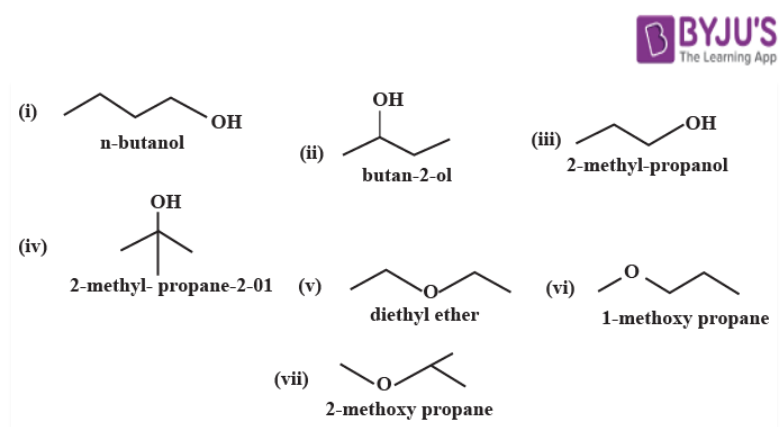Q9:

Define ionic bond. Explain the factors that influence the formation of an Ionic bond with suitable examples.

The force of attraction between oppositely charged ions in a chemical molecule creates an ionic bond, also known as an electrovalent bond. When one atom’s valence (outer) electrons are permanently transferred to another atom, this type of bond is established. The atom that releases electrons becomes a positively charged ion (cation), while the atom that obtains them becomes a negatively charged ion (anion).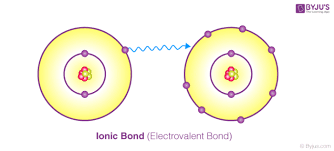Ionic bonding produces ionic, or electrovalent, compounds, such as those generated between non-metals and alkali and alkaline-earth metals. The ions in these ionic crystalline solids are organised in such a way that the positive and negative charges alternate and balance one another, with the total charge of the substance remaining zero.

The formation of ionic bonds is primarily influenced by three factors. They are as follows:

(i) Ionisation energy:

It is the amount of energy necessary to remove the most loosely attached electron from an element’s isolated gaseous atom. The easier it is to create a cation when the ionisation energy is low.

(ii) Electron affinity:

When an electron is added to an isolated gaseous atom of an element, the quantity of energy released is specified. The easier it is to generate an anion, the more energy is released during this process.

Thus, a metal atom’s low ionisation energy and a nonmetal atom’s strong electron affinity encourage the creation of an ionic bond between them.

(iii) Lattice energy:

The amount of energy released when cations and anions are moved from infinity to their respective equilibrium positions in the crystal lattice to create one mole of the ionic compound is defined as lattice energy. The greater the tendency for an ionic bond to form, the higher the lattice energy. The greater the force of attraction between the ions, the higher the charges on the ions and the smaller the space between them.

Q10:

What are quantum numbers? What permitted values can these have? Explain their significance.

Quantum numbers are a set of numbers that represent the position and energy of an electron in an atom. The state of an electron in an atom or ion is defined by four quantum numbers, which yield solutions to the Schrödinger wave equation for the hydrogen atom.

There are four quantum numbers:

(i) ‘n’ is the principal quantum number. The quantity of the electron’s shell is the principal quantum number, which is an integer. The quantity is one or more (never 0 or negative).

(ii) ‘l is orbital angular momentum quantum number (or azimuthal quantum number. The orbital’s form is defined by the angular quantum number (l). Spherical (l = 0), polar (l = 1), or cloverleaf (l = 2) are the best descriptions for orbitals. As the angular quantum number grows greater, they can take on increasingly complicated shapes.

(iii) Magnetic quantum number (ml or m): characterises the subshell orbital. Having integer values range from -l to l, the magnetic quantum number is the orbital’s orientation. As an outcome, for the p orbital, where l=1, m might be -1, 0, or 1.

(iv) ‘ms’ stands for the electron spin quantum number. The spin quantum number is a half-integer quantity that is either -1/2 (referred to as “spin down”) or 1/2 (referred to as “spin-up”) (called “spin up”).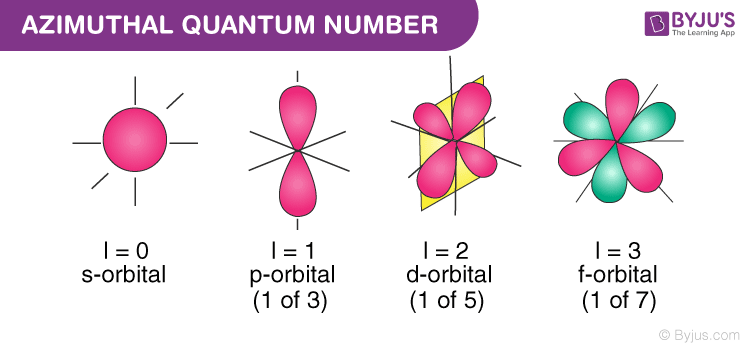Q11:

a) Convert :

i) Benzene to p-nitrobromobenzene

ii) Ethyl chloride to ethene.

b) Give mechanism of addition of HBr to propene.

c) Write a note on Friedel-Crafts alkylation

(a)

1. i) Converting Benzene to p-nitrobromobenzene:

Benzene can be transformed to p-nitrobromobenzene through electrophilic aromatic substitution with bromine in the presence of ferric bromide. Fractional distillation separates ortho-bromonitrobenzene and para-bromo nitrobenzene after nitration with concentric nitric acid and concentric sulphuric acid.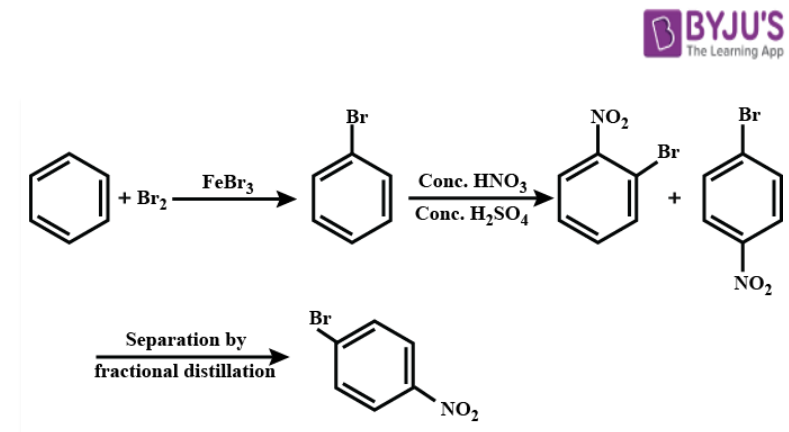ii) Converting Ethyl chloride to ethene:

Conversion of Ethyl chloride to ethene is an Elimination reaction.

C2H5Cl + KOH (alcoholic soln.) → CH2 = CH2 + HCl

1. Mechanism of addition of HBr to propene.

Step 1: Electrophile formation

Br is more electronegative than H in HBr. Polarity is created when bound electrons travel towards Br, resulting in the formation of electrophile H+, which attacks the double bond to form a carbocation.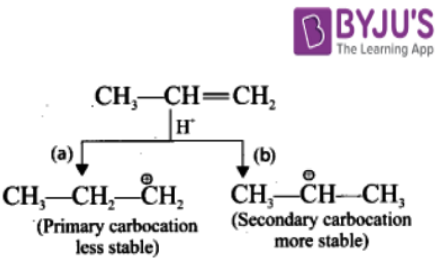Step 2:

Secondary carbocation is more stable than primary carbocation, and it takes priority over it.

Step 3:

The Br-1 ion attacks the 2°- carbocation, resulting in the formation of 2- Bromo propane as the main product.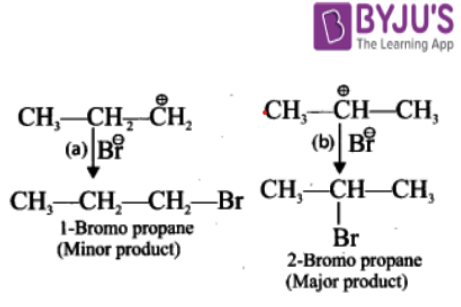(c) Note on Friedel-Crafts Alkylation:

Friedel-Crafts reactions are organic coupling reactions that use an electrophilic aromatic substitution to attach substituents to aromatic rings. Alkylation and acylation are the two main forms of Friedel-Crafts processes. French chemist Charles Friedel and American chemist James Crafts invented these reactions in 1877.

An aromatic proton is replaced with an alkyl group in Friedel-Crafts alkylation. This is accomplished by using a carbocation to perform an electrophilic assault on the aromatic ring. Using alkyl halides as reactants, the Friedel-Crafts alkylation process produces alkylbenzenes.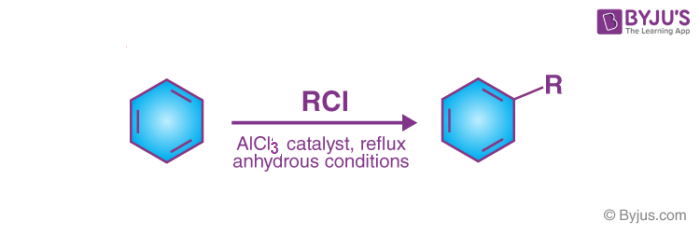This reaction can’t utilise the carbocations generated by aryl and vinyl halides because they’re too unstable. To avoid polyalkylation in these processes, a large amount of the aromatic component must be utilised (addition of more than one alkyl group to the aromatic compound).

The Friedel-Crafts alkylation reaction does not include aromatic compounds that are less reactive than mono-halobenzenes. It’s important to remember that this reaction, like any other involving carbocations, is susceptible to carbocation rearrangements.

Friedel Crafts Alkylation And Acylation

Q12:

Balance the following equation in the acidic medium by the half-reaction method.

Cr2O72- + C2H4O → C2H4O2 + Cr3+

Adding electrons to whatever side is required to make up the difference in oxidation number in each half-reaction is known as the half-reaction method or ion-electron method. If the reaction takes place in an acidic media, the charge is balanced by introducing H+ ions. If the reaction takes place in basic media, add OH ions.

To begin, balance the equations in terms of electrons.

Reduction:

Cr2O72- + 6e = 2 Cr3+

Oxidation:

C2H4O = C2H4O2 + 2e………. Multiply this reaction by 3 to get the final result

In the oxidation process, the oxygen atoms are not balanced. To counteract this, add as many water molecules to the right as the total oxygen to the left requires. Then, on the left side of the equation, add hydrogen ions to balance the number of hydrogen atoms injected with the water molecules.

Cr2O72- + 14 H+ + 6e = 2 Cr3+ + 7 H2O

3C2H4O + 3H2O = 3C2H4O2 + 6H+ + 6e

By combining the two half-reactions, the electrons on either side of the equation are now equal.

Cr2O72- + 3 C2H4O + 8H+ = 2 Cr3+ + 3 C2H4O2 + 4 H2O

Q13:

(a) Give one method for industrial preparation and one for laboratory preparation of CO and CO2 each.

(b) Select the member(s) of group 14 that

i) form the most acidic dioxide

ii) are used as semiconductors.

(c) Explain the structure of Diborane

(a) Laboratory preparation of carbon monoxide (CO):

At 373, concentrated sulphuric acid is used to dehydrate formic acid.

$$\begin{array}{l}HCOOH \xrightarrow[conc H_{2}SO_{4}]{373K} H_{2}O + CO↑\end{array}$$

Industrial preparation of CO:

When steam is passed over hot coke, carbon monoxide is produced.

$$\begin{array}{l}C + H_{2}O \xrightarrow[]{473-1273K} CO + H_{2}\end{array}$$

(water gas)

Industrial preparation of carbon dioxide (CO2):

Carbon dioxide is produced by heating limestone.

$$\begin{array}{l}CaCO_{3} \xrightarrow[]{Heat} CaO + CO_{2}\end{array}$$

Carbon dioxide preparation in the laboratory:

Carbon dioxide is formed when calcium carbonate combines with dilute HCl.

CaCO3 +2HCl → CaCl2 + CO2 + H2O

(b)

1. i) Because carbon is the most non-metallic element in group 14, its dioxide is the most acidic.

ii) Silicon and germanium are used as semiconductors.

(c) The chemical compound diborane has the molecular formula B2H6 and is made up of boron and hydrogen atoms. This chemical has a sweet odour and is highly unstable at room temperature. Boranes are chemicals that include both boron and hydrogen atoms. One of most basic boron hydrides is diborane.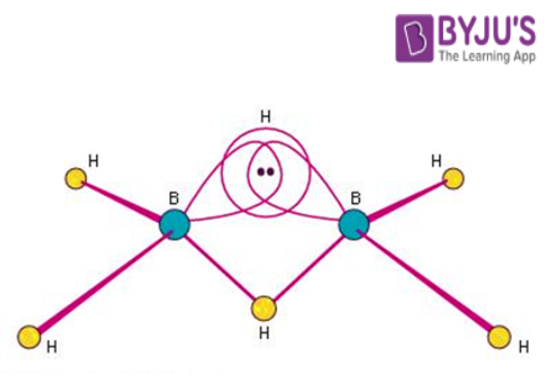The Diborane molecule is made up of four hydrogen atoms and two boron atoms that are all in the same dimension. Two separating hydrogen atoms are known to exist between these planes.

Boron possesses four hybrid orbitals and is known to be sp3 hybridised. Three of the four hybrid orbitals have one electron apiece, while the fourth orbital is empty. Each boron atom’s two hybrid orbital electrons make two bonds with hydrogen atoms in the 1s position. The banana bond is formed by the two boron atoms remaining with each unpaired electron orbital and empty orbital forming two bridgings (B–H–B) bonds with the two 1s hydrogen atoms.

Q14:

Convert the following:

a) Benzene to Benzoic acid

b) Bromoethane to Butan-1-ol

c) Ethene to Propene

d) Ethyne to Methane

e) Propene to Propan-2-ol

(a) Benzene to Benzoic acid

$$\begin{array}{l}C_{6}H_{6} \xrightarrow[CH_{3}Cl]{AlCl_{3}} C_{6}H_{5}-CH_{3}\xrightarrow[]{KMnO_{4}} C_{6}H_{5}COOH\end{array}$$

By first turning benzene to toluene via Freidel Craft’s alkylation reaction, benzene can be converted into benzoic acid.

To produce benzoic acid, toluene is oxidised in the presence of an oxidising agent such as potassium permanganate.

(b) Bromoethane to Butan-1-ol

• First, bromoethane is burned with Na in dry ether to produce n-butane, a higher alkane.
• Then, in the presence of UV light, n-butane is heated with chlorine. After that, we have chlorobutane.
• Butan-1-ol is formed when chlorobutane is treated with NaOH.

C4H9Cl + NaOH → C4H9OH + NaCl

(c)Ethene to Propene

To convert ethene to propene, we need to increase one carbon in the chain because ethene has 2 carbons whereas propene has 3 carbons.

CH2=CH2 → CH3-CH=CH2

Alkyl halide (HBr) is the mother compound to increase carbon in a chain.

Ethene will react with HBr and we will get 1-bromoethane.

$$\begin{array}{l}CH_{2}= CH_{2} \overset{HBr}{\rightarrow} CH_{3}-CH_{2}-Br\end{array}$$

1-bromoethane is treated with KCN to give propane nitrile.

$$\begin{array}{l}CH_{3}-CH_{2}-Br \overset{KCN}{\rightarrow} CH_{3}-CH_{2}-CN\end{array}$$

Propane nitrile is then converted to propylamine when treated with LiAlH4.

$$\begin{array}{l}CH_{3}-CH_{2}-CN \xrightarrow[]{LiAlH_{4}} CH_{3}-CH_{2}-CH_{2}-NH_{2}\end{array}$$

When treated with NaNO2 and HCl, propylamine is converted to propanol.

$$\begin{array}{l}CH_{3}-CH_{2}-CH_{2}-NH_{2} \xrightarrow[> 10C]{NaNO_{2},HCl} CH_{3}-CH_{2}-CH_{2}-OH\end{array}$$

Propanol produces 1-chloropropane when treated with PCl5.

$$\begin{array}{l}CH_{3}-CH_{2}-CH_{2}-OH \xrightarrow[]{PCl_{5}} CH_{3}-CH_{2}-CH_{2}-Cl\end{array}$$

Finally, 1-chloropropane produces propene when treated with KOH.

$$\begin{array}{l}CH_{3}-CH_{2}-CH_{2}-Cl \xrightarrow[]{KOH} CH_{3}-CH= CH_{2}\end{array}$$

(d)Ethyne to Methane

This conversion is broken down into four steps.

Step 1: When ethyne reacts directly with water in the presence of mercury(II) salts, the result is ethanal.

$$\begin{array}{l}HC\equiv CH + H_{2}O \xrightarrow[catalyst]{Hg^{2+}} H_{3}C-CHO\end{array}$$

Step 2: By using acid dichromate as an oxidising agent, ethanal oxidises to ethanoic acid.

$$\begin{array}{l}H_{3}C-CHO \overset{[O]}{\rightarrow} H_{3}C-COOH\end{array}$$

Step 3: Now, ethanoic acid reacts with NaOH and vaporises to dryness before being heated gently to produce anhydrous sodium acetate.

$$\begin{array}{l}H_{3}C-COOH + NaOH \to H_{3}C-COONa^{+} + H_{2}O\end{array}$$

Step 4: The methane is then produced by heating anhydrous sodium acetate with soda lime.

$$\begin{array}{l}H_{3}C-COONa^{+} + NaOH \to CH_{4} + Na_{2}CO_{3}\end{array}$$

(e)Propene to Propan-2-ol

Propene is converted to propan–2–ol when it is treated with water in the presence of an acid catalyst.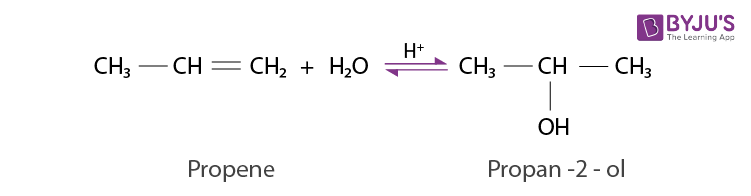Q15:

Write the balanced ionic equation for the reaction of permanganate ion with bromide ion in a basic medium to give manganese dioxide and bromate ion.

Manganese dioxide and bromate ion are formed when permanganate ion combines with bromide ion in a basic media. The balanced chemical equation is:

$$\begin{array}{l}2MnO_{4}^{-}(aq) + Br^{-}(aq) + H_{2}O(l) \to 2MnO_{2}(s) + BrO_{3}^{-}(aq) + 2OH^{-}(aq)\end{array}$$

The ion-electron technique is used to obtain the final reaction. Another way for balancing reactions is to use the oxidation number method.

The oxidation number method involves the following steps:

Step 1: The skeletal equation for the given reaction is: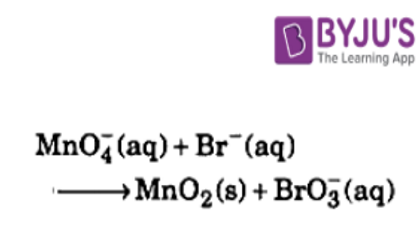Step 2: Determine which elements experience an oxidation number change (O.N.). Per Br atom, O.N. increases by 6.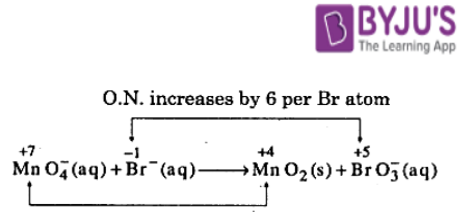Per Mn atom, O.N. falls by three………. (i)

Because the O.N. of Br in BrO3 increases from – 1 Br to +5, Br serves as a reductant. MnO4 serves as an oxidant because the O.N. of Mn falls from +7 in MnO4 to +4 in MnO2.

Step 3: H2O molecules are used to balance out the O atoms.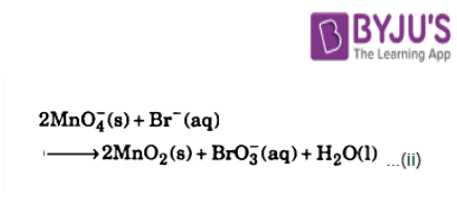Step 4: Because the reaction happens in basic media, we can balance H atoms by adding H2O and OH ions. By adding 2H2O to L.H.S. and 2OH to R.H.S. of Eq. (ii), we get: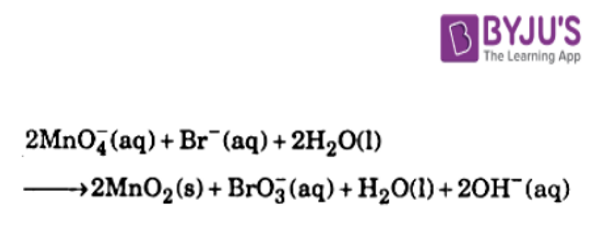Test your Knowledge on Important 5 marks questions for cbse class 11 chemistry!